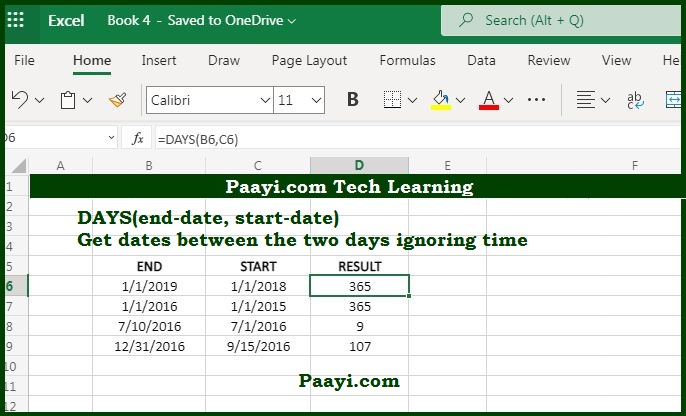# Learn How to Use Microsoft Excel DAYS Function

Written by | 0 Comments | 654 Views

In this article, you will learn how to use the Microsoft Excel DAYS function and its prime function in Microsoft Excel. You will also get to know the Microsoft Excel DAYS function return value and syntax with the help of some examples.

## Microsoft Excel DAYS Function

The main purpose of the DAYS function is to get the days between the days. So, the Microsoft Excel DAYS function returns the number of days between the two dates. If you want to use this function as a start date in A1 and end date in B1, you need to use - = DAYS(B1, A1), and this will return the days between the two specified dates.

### DAYS Function Return Value

It will be the number representing the days between two specified dates.

### DAYS Function Syntax

= DAYS(end-date, start-date)

Where:

• end-date: It is the end date.
• start-date: It is the start date.

How to Use the Microsoft Excel DAYS Function?as we know, the DAYS function returns the number of dates between the two specified dates. If you want to use it as a start date between A1 and end date as A2, the syntax will be:

= DAYS(A1,A2)